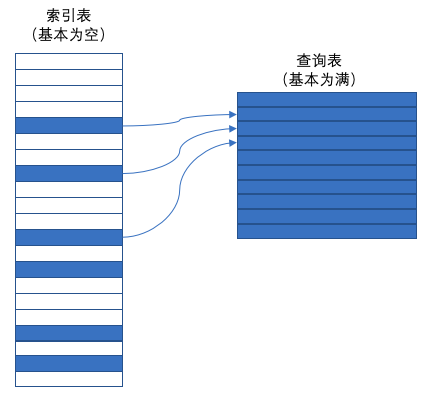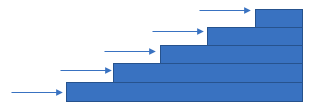# 一文道尽“表驱动法”## 使用总则

﻿

﻿

if ((( 'a' <= inputChar ) && ( inputChar <= 'z' )) || (( 'A' <= inputChar ) && ( inputChar <= 'Z' ))){    charType = CharacterType.Letter;} else if  (( inputChar = '' ) || ( inputChar = ',' ) || ( inputChar = '.' ) || ( inputChar = '!' ) || ( inputChar = '(' ) || ( inputChar = ')' ) || ( inputChar = ':' ) || ( inputChar = ';' ) || ( inputChar = '?') || ( inputChar = '-' )) {    charType = CharacterType.Punctuation;} else if (( '0' <= inputChar && inputChar <= '9' )) {    charType = CharacterType.Digit;}

charType = charTypeTable[inputChar];

## 使用表驱动法的两个问题

1）如何从表中查数据？

• 直接访问

• 索引访问

• 阶梯访问

2）在表里存些什么？

• 数据

• 动作（action）-描述该动作的代码/该动作的子程序的引用。

### 1、直接访问

﻿

﻿

if(month == 1) {    days = 31;} else if (month = 2){    days = 28;} else if (month = 3){    days = 31;} else if (month = 4){    days = 30;} else if (month = 5){    days = 31;} else if (month = 6){    days = 30;} else if (month = 7){    days = 31;} else if (month = 8){    days = 31;} else if (month = 9){    days = 30;} else if (month = 10){    days = 31;} else if (month = 11){    days = 30;} else if (month = 12){    days = 31;}

[31, 28, 31, 30, 31, 30, 31, 31, 30, 31, 30, 31]

charType = charTypeTable[inputChar];

#### 1）复制信息从而能够直接使用键值

使age能像键值一样用于费率表的种查询方法是将1-17岁之间的年龄都复制一份18岁以下的费率，然后直接用该age键值来访问表。同样也可以用同样的方法来处理66岁以上的情况。

﻿

#### 2）转换键值以使其能够直接使用

使age能像键值一样用于费率表查询的第二个方法是用一个函数将age转换为另一个数值。在此例子中，该函数必须把所有介于1-17直接的年龄转换成一个键值，例如17，同时把所有超过66的年龄都转换成另一个键值，例如66。

﻿

#### 3）把键值转换提取城独立子程序

如果你必须要构造一些数据来让它们像表键值一样使用，那就把数据到键值的转换操作提取成独立的子程序。这样可避免在不同位置执行了不同的转换，也使得转换操作修改起来更加容易。

﻿

### 2、索引访问

﻿

﻿

﻿• 如果主查询表的每条记录都很大，那创建一个浪费了很多空间的数组所用的空间，要比建立主查询表所用的空间小得多。

• 操作索引中的记录比操作主查询表的的记录更方便，编写到表里面的数据比嵌入代码的数据更容易维护。

﻿

### 3、阶梯访问

﻿﻿

>= 90.0%      A

<90.0%         B

<75.0%         C

<65.0%         D

<50.0%         F

﻿

﻿

Dim rangeLimit() As Double = {50.0, 65.0, 75.0, 90.0, 100.0}Dim grade() As String={"F", "D", "C", "B", "A"}maxGradeLevel = grade.Length - 1assign a grad to a student based on the student's scoreGradeLevel= 1 StudentGrade = ”A" while( StudentGrade = "A" and GradeLevel < MaxGradeLevel )     if( StudentScore < RangeLimit( GradeLevel ) ) then         StudentGrade = Grade ( GradeLevel)     end if    GradeLevel = GradeLevel + 1 Wend

## 总结

1. 表驱动法提供了一种复杂的逻辑和继承结构的替换方案。如果你发现自己对某个应用程序的逻辑或者继承关系感到困惑，那是否可以通过一个查询表来加以简化。

2. 使用表的关键决策是决定如何去访问表，可以采取直接访问、索引访问或阶梯访问

3. 使用表的另一项关键决策是决定如何去把什么内容放入表中

4. 需要保存浮点数和范围数时，使用阶梯访问的形式。

﻿

﻿### 架构精进之路

「架构精进之路」公号作者 0x0a年互联网从业经验，能编码、做架构、懂管理，坚持总结、沉淀和分享

## 评论